##用户名 Email 自动登录 找回密码 密码 立即注册

# 单片机脉搏体温检测仪的Proteus仿真设计与调试 附源码+文档362443451 发表于 2019-1-10 21:28 | 只看该作者 |只看大图|倒序浏览 |阅读模式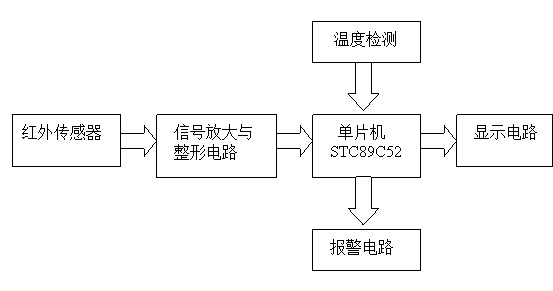2.1主控模块的选型和论证

2.2显示模块的选型和论证

2.3脉搏检测传感器的选型和论证

2.4温度传感器的选型

考虑到在单片机属于数字系统，容易想到数字温度传感器，可选用DS18B20数字温度传感器，此传感器为单总线数字温度传感器，起体积小、构成的系统结构简单，它可直接将温度转化成串行数字信号给单片机处理，即可实现温度显示。另外DS18B20具有3引脚的小体积封装，测温范围为-55~+125摄氏度，测温分辨率可达0.0625摄氏度，其测量范围与精度都能符合设计要求。
以上两种方案相比较，第二种方案的电路、软件设计更简单，此方案设计的系统在功耗、测量精度、范围等方面都能很好地达到要求，故本设计采用方案二。

2.5系统整体设计概述

1、硬件原理图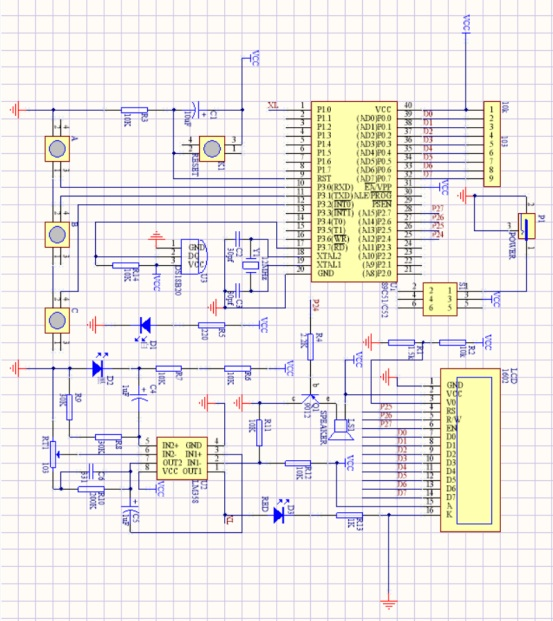2、工作原理

3.1.1单片机最小系统设计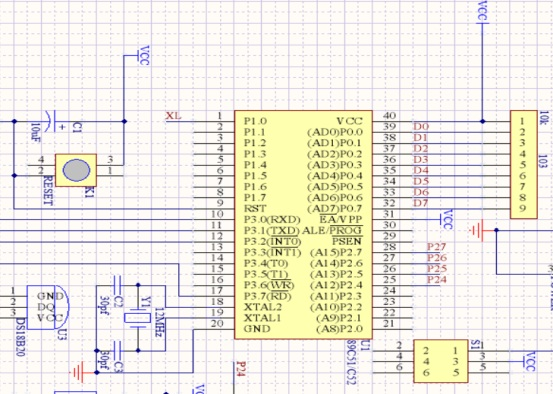图3.1为单片机最小系统电路图，单片机最小系统有单片机、时钟电路、复位电路组成，时钟电路选用了12MHZ的晶振提供时钟，作用为给单片机提供一个时间基准，其中执行一条基本指令需要的时间为一个机器周期，单片机的复位电路，按下复位按键之后可以使单片机进入刚上电的起始状态。图中10K排阻为P0口的上拉电阻，由于P0口跟其他IO结构不一样为漏极开路的结构，因此要加上拉电阻才能正常使用。

3.1.2 液晶原理介绍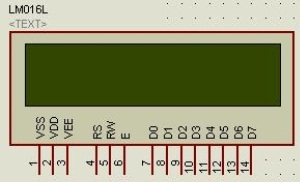LCD1602引脚图

 RS R/W 操作说明 0 0 写入指令寄存器（清除屏等） 0 1 读busy flag（DB7），以及读取位址计数器（DB0~DB6）值 1 0 写入数据寄存器（显示各字型等） 1 1 从数据寄存器读取数据

LCD1602与单片机接口电路如下：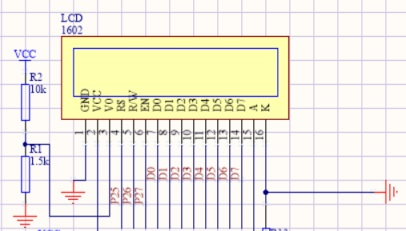3.1.3信号采集电路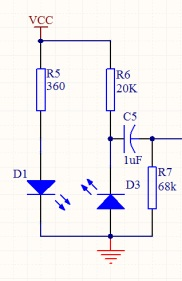3.1.4信号放大电路

图中C7为耦合电容，作用为隔直流通交流，之所以使用1uF的电容，是为了让所有的信号通过。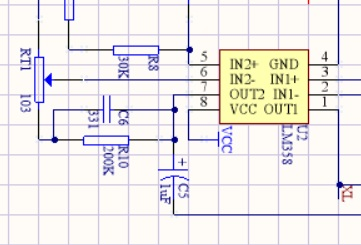3.1.5信号比较电路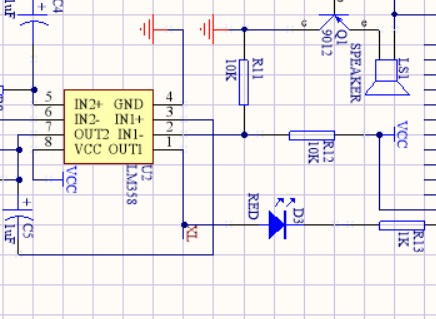3.2系统软件总体设计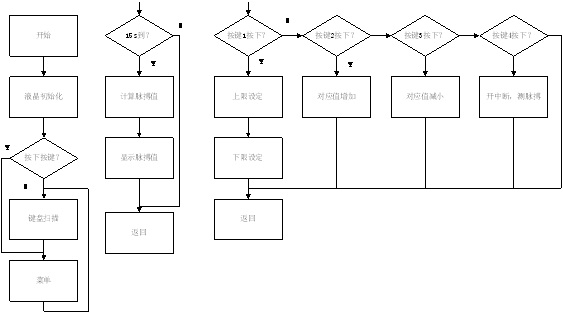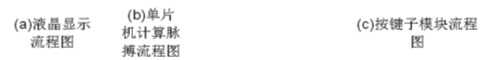4.1软件调试

4.2硬件调试

1. #include <reg52.h>
2. #include <intrins.h>

3. #define uint            unsigned int
4. #define uchar           unsigned char
5. #define ulong           unsigned long         //宏定义
6. #define LCD_DATA        P0                                 //定义P0口为LCD_DATA

7. sbit LCD_RS =P2^5;
8. sbit LCD_RW =P2^6;
9. sbit LCD_E  =P2^7;                                                 //定义LCD控制引脚

10. sbit Xintiao =P1^0 ;                                         //脉搏检测输入端定义
11. sbit speaker =P2^4;                                                 //蜂鸣器引脚定义
12. sbit DQ=P3^7;

13. void delay5ms(void);   //误差 0us
14. void LCD_WriteData(uchar LCD_1602_DATA);         /********LCD1602数据写入***********/
15. void LCD_WriteCom(uchar LCD_1602_COM);                 /********LCD1602命令写入***********/
17. void InitLcd();//液晶初始化函数

18. void Tim_Init();

19. uchar Xintiao_Change=0;           //
20. uint  Xintiao_Jishu;
21. uchar stop;
22. uchar View_Data;
23. uchar View_L;
24. uchar View_H;
25. uchar Xintiao_H=100;        //脉搏上限
26. uchar Xintiao_L=40;                //脉搏下限
27. uint wendu=0;

28. uchar Key_Change;
29. uchar Key_Value;                //按键键值
30. uchar View_Con;                        //设置的位（0正常工作，1设置上限，2设置下限）
31. uchar View_Change;

32. /*****延时子程序*****/
33. void Delay_DS18B20(int num)
34. {
35.   while(num--) ;
36. }
37. /*****初始化DS18B20*****/
38. void Init_DS18B20(void)
39. {
40.   unsigned char x=0;
41.   DQ = 1;         //DQ复位
42.   Delay_DS18B20(8);    //稍做延时
43.   DQ = 0;         //单片机将DQ拉低
44.   Delay_DS18B20(80);   //精确延时，大于480us
45.   DQ = 1;         //拉高总线
46.   Delay_DS18B20(34);
47. }
48. /*****读一个字节*****/
49. unsigned char ReadOneChar(void)
50. {
51.   unsigned char i=0;
52.   unsigned char dat = 0;
53.   for (i=8;i>0;i--)
54.   {
55.     DQ = 0;     // 给脉冲信号
56.     dat>>=1;
57.     DQ = 1;     // 给脉冲信号
58.     if(DQ)
59.     dat|=0x80;
60.     Delay_DS18B20(4);
61.   }
62.   return(dat);
63. }

64. void WriteOneChar(unsigned char dat)
65. {
66.   unsigned char i=0;
67.   for (i=8; i>0; i--)
68.   {
69.     DQ = 0;
70.     DQ = dat&0x01;
71.     Delay_DS18B20(5);
72.     DQ = 1;
73.     dat>>=1;
74.   }
75. }
76. /*****读取温度*****/
77. unsigned int ReadTemperature(void)
78. {
79.   unsigned char a=0;
80.   unsigned char b=0;
81.   unsigned int t=0;
82.   float tt=0;
83.   Init_DS18B20();
84.   WriteOneChar(0xCC);  //跳过读序号列号的操作
85.   WriteOneChar(0x44);  //启动温度转换
86.   Init_DS18B20();
87.   WriteOneChar(0xCC);  //跳过读序号列号的操作
88.   WriteOneChar(0xBE);  //读取温度寄存器
91.   t=b;
92.   t<<=8;
93.   t=t|a;
94.   tt=t*0.0625;
95.   t= tt*10+0.5;     //放大10倍输出并四舍五入
96.   return(t);
97. }
98. //=====================================================================================
99. //=====================================================================================
100. //=====================================================================================

101. void main()          //主函数
102. {
103. InitLcd();
104. Tim_Init();
105. lcd_1602_word(0x80,16,"Heart Rate:     ");          //初始化显示

106. TR0=1;
107. TR1=1;                                  //打开定时器
108. while(1)                          //进入循环
109.   {
110.           if(View_Con==0)
111.         {
113.                 lcd_1602_word(0xc0,10," Temp:    ");//显示第二行数据
114.                 LCD_WriteCom(0x80+0x40+10);
115.                 LCD_WriteData(wendu/100+0x30);
116.                 LCD_WriteData(wendu%100/10+0x30);
117.                 LCD_WriteData('.');
118.                 LCD_WriteData(wendu%100%10+0x30);
119.                 LCD_WriteData(0xdf);
120.                 LCD_WriteData('C');
121.         }
122.
123.    if(Key_Change)          //有按键按下并已经得出键值
124.     {
125.          Key_Change=0;          //将按键使能变量清零，等待下次按键按下
126.          View_Change=1;
127.          switch(Key_Value)                                //判断键值
128.           {
129.            case 1:                                                //设置键按下
130.                  {
131.                           View_Con++;                        //设置的位加
132.                           if(View_Con==3)                //都设置好后将此变量清零
133.                            View_Con=0;
134.                           break;                                //跳出，下同
135.                          }
136.            case 2:                                                //加键按下
137.                  {
138.                           if(View_Con==2)                //判断是设置上限
139.                            {
140.                                    if(Xintiao_H<150)        //上限数值小于150
141.                                  Xintiao_H++;                //上限+
142.                            }
143.                           if(View_Con==1)                //如果是设置下限
144.                            {
145.                                    if(Xintiao_L<Xintiao_H-1)//下限值小于上限-1（下限值不能超过上限）
146.                                  Xintiao_L++;                //下限值加
147.                            }
148.                           break;
149.                          }
150.            case 3:                                                //减键按下
151.                  {
152.                           if(View_Con==2)                //设置上限
153.                            {
154.                                    if(Xintiao_H>Xintiao_L+1)//上限数据大于下限+1（同样上限值不能小于下限）
155.                                  Xintiao_H--;                //上限数据减
156.                            }
157.                           if(View_Con==1)                //设置下限
158.                            {
159.                                    if(Xintiao_L>30)        //下限数据大于30时
160.                                  Xintiao_L--;                //下限数据减
161.                            }
162.                           break;
163.                          }
164.          }
165.         }
166.    if(View_Change)//开始显示变量
167.     {
168.          View_Change=0;//变量清零
169.          if(stop==0)                          //心率正常时
170.           {
171.            if(View_Data==0x30) //最高位为0时不显示
172.             View_Data=' ';
173.           }
174.          else                                          //心率不正常（计数超过5000，也就是两次信号时间超过5s）不显示数据
175.           {
176.            View_Data=' ';
177.            View_Data=' ';
178.            View_Data=' ';
179.           }

180.          switch(View_Con)
181.           {
182.            case 0: //正常显示
183.                   {
184.                            lcd_1602_word(0x80,16,"Heart Rate:     ");//显示一行数据

185.                            lcd_1602_word(0x8d,3,View_Data);                         //第1行显示心率

186.                            break;
187.                           }
188.            case 1: //设置下限时显示
189.                   {
190.                            lcd_1602_word(0x80,16,"Heart Rate:     ");//第一行显示心率
191.                            lcd_1602_word(0x8d,3,View_Data);
192.
193.                            View_L=Xintiao_L/100+0x30;                //将下限数据拆字
194.                            View_L=Xintiao_L%100/10+0x30;
195.                            View_L=Xintiao_L%10+0x30;

196.                            if(View_L==0x30)                                        //最高位为0时，不显示
197.                             View_L=' ';
198.
199.                            lcd_1602_word(0xC0,16,"Warning L :     ");//第二行显示下限数据
200.                            lcd_1602_word(0xCd,3,View_L);
201.                            break;
202.                           }
203.            case 2: //设置上限时显示（同上）
204.                   {
205.                            lcd_1602_word(0x80,16,"Heart Rate:     ");
206.                            lcd_1602_word(0x8d,3,View_Data);
207.
208.                            View_H=Xintiao_H/100+0x30;
209.                            View_H=Xintiao_H%100/10+0x30;
210.                            View_H=Xintiao_H%10+0x30;

211.                            if(View_H==0x30)
212.                             View_H=' ';
213.
214.                            lcd_1602_word(0xC0,16,"Warning H :     ");
215.                            lcd_1602_word(0xCd,3,View_H);
216.                            break;
217.                           }
218.           }
219.         }
220.   }
221. }

222. void Time1() interrupt 3                //定时器1服务函数
223. {
224.         static uchar Key_Con,Xintiao_Con;
225.         TH1=0xd8;                   //10ms
226.         TL1=0xf0;                   //重新赋初值
227.         switch(Key_Con)   //无按键按下时此值为0
228.         {
229.                 case 0:                   //每10ms扫描此处
230.                 {
231.                         if((P3&0x07)!=0x07)//扫描按键是否有按下
232.                         {
233.                                 Key_Con++;                  //有按下此值加1，值为1
234.                         }
235.                         break;
236.                 }
237.                 case 1:                                          //10ms后二次进入中断后扫描此处（Key_Con为1）
238.                 {
239.                         if((P3&0x07)!=0x07)//第二次进入中断时，按键仍然是按下（起到按键延时去抖的作用）
240.                         {
241.                                 Key_Con++;                  //变量加1，值为2
242.                                 switch(P3&0x07)  //判断是哪个按键按下
243.                                 {
244.                                         case 0x06:Key_Value=1;break;         //判断好按键后将键值赋值给变量Key_Value
245.                                         case 0x05:Key_Value=2;break;
246.                                         case 0x03:Key_Value=3;break;
247.                                 }
248.                         }
249.                         else                                                                 //如果10ms时没有检测到按键按下（按下时间过短）
250.                         {
251.                                 Key_Con=0;                                                 //变量清零，重新检测按键
252.                         }
253.                         break;
254.                 }
255.                 case 2:                                                                         //20ms后检测按键
256.                 {
257.                         if((P3&0x07)==0x07)                                 //检测按键是否还是按下状态
258.                         {
259.                                 Key_Change=1;                                         //有按键按下使能变量，（此变量为1时才会处理键值数据）
260.                                 Key_Con=0;                                                //变量清零，等待下次有按键按下
261.                         }
262.                         break;
263.                 }
264.         }
265.
266.         switch (Xintiao_Con)//此处与上面按键的检测类似
267.         {
268.                 case 0:                         //默认Xintiao_Con是为0的
269.                 {
270.                         if(!Xintiao)//每10ms（上面的定时器）检测一次脉搏是否有信号
271.                         {
272.                                 Xintiao_Con++;//如果有信号，变量加一，程序就会往下走了
273.                         }
274.                         break;
275.                 }
276.                 case 1:
277.                 {
278.                         if(!Xintiao)           //每过10ms检测一下信号是否还存在
279.                         {
280.                                 Xintiao_Con++;//存在就加一
281.                         }
282.                         else
283.                         {
284.                                 Xintiao_Con=0;//如果不存在了，检测时间很短，说明检测到的不是脉搏信号，可能是其他干扰，将变量清零，跳出此次检测
285.                         }
286.                         break;
287.                 }
288.                 case 2:
289.                 {
290.                         if(!Xintiao)
291.                         {
292.                                 Xintiao_Con++;//存在就加一
293.                         }
294.                         else
295.                         {
296.                                 Xintiao_Con=0;//如果不存在了，检测时间很短，说明检测到的不是脉搏信号，可能是其他干扰，将变量清零，跳出此次检测
297.                         }
298.                         break;
299.                 }
300.                 case 3:
301.                 {
302.                         if(!Xintiao)
303.                         {
304.                                 Xintiao_Con++;//存在就加一
305.                         }
306.                         else
307.                         {
308.                                 Xintiao_Con=0;//如果不存在了，检测时间很短，说明检测到的不是脉搏信号，可能是其他干扰，将变量清零，跳出此次检测
309.                         }
310.                         break;
311.                 }
312.                 case 4:
313.                 {
314.                         if(Xintiao)//超过30ms一直有信号，判定此次是脉搏信号，执行以下程序
315.                         {
316.                                 if(Xintiao_Change==1)//心率计原理为检测两次脉冲间隔时间计算心率，变量Xintiao_Change第一次脉冲时为0的，所有走下面的else，第二次走这里
317.                                 {
318.                                         View_Data=(60000/Xintiao_Jishu)/100+0x30;
319.                                         View_Data=(60000/Xintiao_Jishu)%100/10+0x30;
320.                                         View_Data=(60000/Xintiao_Jishu)%10+0x30;
321.
322.                                         if(((60000/Xintiao_Jishu)>=Xintiao_H)||((60000/Xintiao_Jishu)<=Xintiao_L))//心率不在范围内报警
323.                                         speaker=0;                        //蜂鸣器响
324.                                         else
325.                                         speaker=1;                        //不响
326.
327.                                         View_Change=1;           //计算出心率后启动显示
328.                                         Xintiao_Jishu=0;           //心跳计数清零
329.                                         Xintiao_Change=0;   //计算出心率后该变量清零，准备下次检测心率
330.                                         stop=0;                           //计算出心率后stop清零
331.                                    TR0=0;
333.                                 //   TR1=0;
334.                                 }
335.                                 else//第一次脉冲时Xintiao_Change为0
336.                                 {
337.                                         Xintiao_Jishu=0;        //脉冲计时变量清零，开始计时
338.                                         Xintiao_Change=1;//Xintiao_Change置1，准备第二次检测到脉冲时计算心率
339.                                         TR0=1;
340.                                 }
341.                                 Xintiao_Con=0;        //清零，准备检测下一次脉冲
342.                                 break;
343.                         }
344.                 }
345.         }
346. }
347. /**定时器T0工作函数**/
348. void Time0() interrupt 1
349. {
350. TH0=0xfc;                   //1ms
351. TL0=0x18;                   //重新赋初值
352. Xintiao_Jishu++;  //心跳计数加
353. if(Xintiao_Jishu==5000)//心跳计数大于5000
354.   {
355.    Xintiao_Jishu=0;                //数据清零
356.    View_Change=1;                //显示位置1
357.    Xintiao_Change=0;        //置零，准备再次检测
358.    stop=1;           //心跳计数超过5000后说明心率不正常或者没有测出，stop置1
359.    speaker=1;  //关闭蜂鸣器
360.   }
361. }
362. /**定时器初始化函数**/
363. void Tim_Init()
364. {
365. EA=1;                          //打开中断总开关
366. ET0=1;                          //打开T0中断允许开关
367. ET1=1;                          //打开T1中断允许开关
368. TMOD=0x11;                  //设定定时器状态
369. TH0=0xfc;                   //1ms
370. TL0=0x18;                   //赋初值

371. TH1=0xd8;                   //10ms
372. TL1=0xf0;                   //赋初值
373. }

376. {
377. uchar a=0;
378. uchar Data_Word;
381.   {
383.    LCD_WriteData(Data_Word);  //显示字符串
385.   }
386. }

387. ……………………

388. …………限于本文篇幅 余下代码请从51黑下载附件…………16 脉搏体温检测仪的设计与制作2016111435朱波.zip (731.88 KB, 下载次数: 8)

### 评分收藏1转播分享淘帖admin 发表于 2019-1-11 17:02 | 只看该作者
 好资料，51黑有你更精彩!!!

 本版积分规则 回帖并转播 回帖后跳转到最后一页